# A Simple Method of Finding Area of an Ellipse and Volume of an Ellipsoid: A Quick Trick

Knoji reviews products and up-and-coming brands we think you'll love. In certain cases, we may receive a commission from brands mentioned in our guides. Learn more.
Finding area of an ellipse is a very cumbersome task using conventional calculus but using some trick that can be done very easily. In this article I have shown how to do that. The method makes use of the natural length scale inherent in the physical prob

Every physical problem consists of natural scale of units of the parameters involved in it, in terms of which, when the problem is expressed, takes the simplest form and wonderful results can then be obtained very easily. In this article we will use this idea to determine first, area of an ellipse and then volume of an ellipsoid.

Area of an ellipse:

Finding area of an ellipse is a very standard question in calculus, which most of us do not like to do due to cumbersome mathematics involved in the process, but wouldn’t it be great if we could find the result merely by calculating area of a circle? Certainly, yes! All of us love to solve problems which have symmetries, since symmetry makes problems easier. We are exactly going to do that. Before we proceed, here is some recapitulation of the conventional method.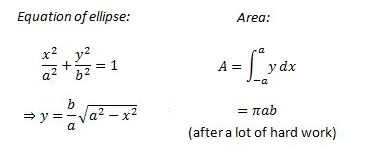Now we shall learn the shortcut techniques.

1. Analytic approach

In this approach we will try to visualize the method graphically. Suppose initially units along x and y axes are given in units of cm. Now if we scale down the units along x axis by a factor of a, the new units become 1/a cm and do the similar along y axis so that new units along y axis become 1/b cm. Then in terms of these units the ellipse becomes a circle with a radius =1. This is shown below.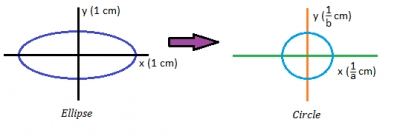Then the area of the circle becomes (PI)*1*1, but this result is obtained in the reduced scale. So if we want to go back to our original length scale then we need to increase the units along x axis by a factor of a and along y axis by a factor b. Hence the area of the original ellipse simply becomes (PI)*a*b.

2. Mathematical approach

In this approach we shall use calculus and the method of change of variables. First we shall make a proper substitution to make the ellipse a circle of radius r. Then calculate the area of the circle which will be obviously (PI)*r*r and this will lead to our required result. All the steps are shown below.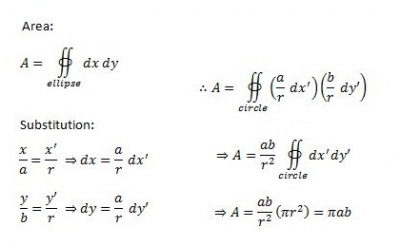Thus we see how easily the result is obtained.

Volume of an ellipsoid:

Exactly same idea can be applied to obtain the volume of an ellipsoid.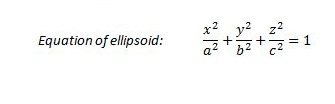1. Analytic approach:

First reduce the units along x, y and z axes by a factor of a, b and c respectively to obtain a sphere whose volume will be (4/3)(PI)*1*1*1 and then scale up each unit to get, volume = (4/3)(PI)*a*b*c.

2. Mathematical Approach

As previously done we shall use the method of substitution to find the result. The steps are shown below.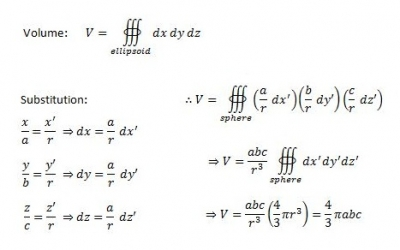Thus we see that how easily the integrals can be performed using these substitutions making use of the inherent natural units of the problems.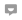aenugu vishwanath reddy
0
Posted on Apr 7, 2012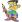roytanay
0
Posted on Apr 3, 2012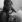DeviPriya Maharana
0
Posted on Apr 3, 2012